# SICP 103 - 构造数据抽象

2021/10/31

Hermann Weyl 在《思维的数学方式》中提到：现在到了数学抽象中最关键的一步：让我们忘记这些符号所表示的对象。.. 数学家不应该在这里停步，有许多操作可以应用于这些符号，而根本不必考虑它们到底代表着什么东西。这句话简要的概括出了编程中“数据抽象”的主要目的：创建一种不依赖特定数据类型的过程，使其可以满足更多不同的用例。

## 数据抽象导引

``````;抽象数据的构造函数和选择函数
(define (make-rat n d)
(define (gcd x y)
(if (= y 0) x (gcd y (remainder x y))))
(cond ((or (and (< n 0) (< d 0)) ;双负得正
(and (> n 0) (< d 0))) ;母负转子负	这个桥梁一般在初始化此抽象数据、定义底层交互时使用，比如这里为 rat 数据抽象定义的 add, sub, mul, div, equal运算符。而之后的过程，则完全基于此抽象之上进行：不论是初始化、调用这些基于 rat 数据抽象的过程都是如此，复合数据背后的实现：cons、car、cdr 均不可见。
(make-rat (- n) (- d)))
(else (let ((g (gcd n d)))
(cons (/ n g) (/ d g))))))
(define (number x) (car x))
(define (denom x) (cdr x))
;基于抽象数据的过程定义
(make-rat (+ (* (number x) (denom y))
(* (number y) (denom x)))
(* (denom x) (denom y))))
(define (sub-rat x y)
(make-rat (- (* (number x) (denom y))
(* (number y) (denom x)))
(* (denom x) (denom y))))
(define (mul-rat x y)
(make-rat (* (number x) (number y))
(* (denom x) (denom y))))
(define (div-rat x y)
(make-rat (* (number x) (denom y))
(* (denom x) (number y))))
(define (equal-rat? x y)
(= (* (number x) (denom y))
(* (number y) (denom x))))
(define (printr x)
(printf "~d/~d\n" (number x) (denom x)))
;基于抽象数据的过程
(define one-half (make-rat 1 2))
(define one-third (make-rat 1 3))
(printr one-half)
(printr one-third)
(printr (mul-rat one-half one-half))
``````

``````(define (make-point x y) (cons x y))
(define (x-point p) (car p))
(define (y-point p) (cdr p))
(define (printp p) (printf "(~d,~d)" (x-point p) (y-point p)))

(define (make-segment x y) (cons x y))
(define (start-segment s) (car s))
(define (end-segment s) (cdr s))
(define (prints s) (printf "[~s,~s]"
(printp (start-segment s))
(printp (end-segment s))))

(define (midpoint-segment s)
(let ((ss (start-segment s))
(se (end-segment s)))
(let ((ssx (x-point ss))
(ssy (y-point ss))
(sex (x-point se))
(sey (y-point se)))
(make-point
(/ (+ ssx sex) 2)
(/ (+ ssy sey) 2)))))
(printp (midpoint-segment (make-segment
(make-point 10 20) (make-point 30 40))))
``````

``````(define (make-rect s1 s2) (cons s1 s2))
(define (h-length r) (define s (car r))
(- (x-point (end-segment s)) (x-point (start-segment s))))
(define (v-length r) (define s (cdr r))
(- (y-point (start-segment s)) (y-point (end-segment s))))

(define (rect-length r)
(* 2 (+ (h-length r) (v-length r))))
(define (rect-area r)
(* (h-length r) (v-length r)))

;重新基于对角 point 实现 rect，应用 rect-length 和 rect-area 完全无需更改即可工作
(define (make-rect p1 p2) (cons p1 p2))
(define (h-length r) (define p1 (car r)) (define p2 (cdr r))
(- (x-point p2) (x-point p1)))
(define (v-length r) (define p1 (car r)) (define p2 (cdr r))
(- (y-point p1) (y-point p2)))
``````

``````(define (cons-car-cdr-1)
(define (cons x y)
(define (dispatch m)
(cond ((= m 0) x)
((= m 1) y)
(else (error "Argument not 0 or 1" m))))
dispatch)
(define (car z) (z 0))
(define (cdr z) (z 1)) 1)
``````

``````(define (cons-car-cdr-2)
(define (cons x y)
(lambda (m) (m x y)))
(define (car z)
(z (lambda (p q) p))) ;((lambda (m) (m x y)) (lambda (p q) p))
(define (cdr z)
(z (lambda (p q) q)))
(newline))

(define (cons-car-cdr-3)
(define (pow a x)
(cond ((= x 0) 1)
((= x 1) a)
(else (* a (pow a (- x 1))))))
(define (loop number)
(lambda (target)
(define (inner-loop now sum)
(cond ((= (remainder now number) 0)
(inner-loop (/ now number) (+ sum 1)))
(else sum)))
(inner-loop target 0)))
(define (cons a b) (* (pow 2 a) (pow 3 b)))
(define (car x) ((loop 2) x))
(define (cdr x) ((loop 3) x))
(printf "cons(5,10) is ~d, car is ~d, cdr is ~d\n"
(cons 5 10) (car (cons 5 10)) (cdr (cons 5 10))))
``````

``````(define zero (lambda (f) (lambda (x) x)))
(lambda (f)
(lambda (x)
(f ((n f) x)))))
;通过代入演算很容易得出 1 2 3 的表示形式
(define one (lambda (f) (lambda (x) (f x))))
(define two (lambda (f) (lambda (x) (f (f x)))))
(define three (lambda (f) (lambda (x) (f (f (f x))))))
;要实现这个过程并不难，只需要将 a 和 b 通过 add-1 的方式表示出来即可
(lambda (a)
(lambda (b)
(lambda (f)
(lambda (x)
((a f) ((b f) x)))))))
``````

## 层次性数据和闭包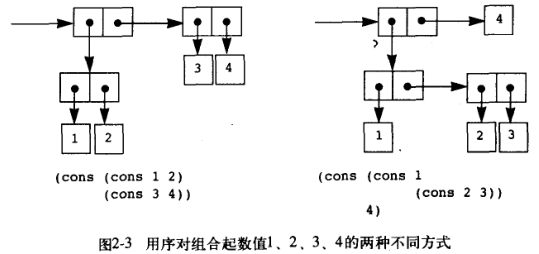### 表的抽象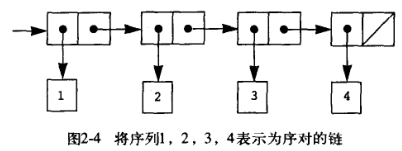``````(define a (list 1 2 3 4));1 2 3 4
(define first-a (car a));1
(define no-head-a (cdr a));2 3 4
(define b (cons "hello" no-head-a)) ;hello 2 3 4
``````

``````(define (item lists n)
(if (= n 0) (car lists) (item (cdr lists) (- n 1))))
(define (size lists)
(if (null? lists) 0 (+ 1 (size (cdr lists)))))
(define (append list1 list2)
(if (null? list1) list2 (cons (car list1) (append (cdr list1) list2))))
(define (last-pair lists)
(define (inner first next)
(if (null? next) (cons first '()) (inner (car next) (cdr next))))
(cond ((null? lists) lists)
((null? (cdr lists)) lists)
(else (inner (car lists) (cdr lists)))))
(define (reverse-err lists)
(if (null? (cdr lists))
(car lists)
(cons (reverse-err (cdr lists)) (car lists))))
(define (reverse-err-2 lists)
(if (null? lists)
'()
(cons (car lists) (reverse-err-2 (cdr lists)))))
(define (reverse lists)
(define (inner remain new)
(if (null? remain) new (inner (cdr remain) (cons (car remain) new))))
(inner lists '()))
``````

``````(define us-coins (list 50 25 10 5 1))
(define uk-coins (list 100 50 20 10 5 2 1 0.5))
(define cn-coins (list 100 50 20 10 5 1))
(define (cc amount coin-values)
(define (no-more? cv) (null? cv))
;基于递归实现(结果反向)
(define (except-first-denomination cv)
(define exclude (first-denomination cv))
(define (inner check collect)
(if (null? check)
collect
(let ((now-check (car check)))
(if (= exclude now-check)
(inner (cdr check) collect)
(inner (cdr check) (cons now-check collect))))))
(inner cv '()))
;基于迭代实现
(define (except-first-denomination cv)
(define exclude (first-denomination cv))
(define (inner check)
(if (null? check) '()
(let ((now-check (car check)))
(if (= exclude now-check)
(inner (cdr check))
(cons now-check (inner (cdr check)))))))
(inner cv))
(define (first-denomination cv)
(let ((now (car cv)))
(if (null? (cdr cv))
now
(if (> now (first-denomination (cdr cv)))
now
(first-denomination (cdr cv))))))
(cond ((= amount 0) 1)
((or (< amount 0) (no-more? coin-values)) 0)
(else
(+ (cc amount
(except-first-denomination coin-values))
(cc (- amount
(first-denomination coin-values))
coin-values)))))
(printf "cc 100 for cn ~d\n" (cc 100 cn-coins))
``````

Scheme 支持可变参数，即将多个连续参数收集到一张表中，使用 x . y 记号，这样传入 1 2 3 4 则 x 为 1，(2 3 4) 为 y。下面是一个基于可变参数实现的过滤和第一个参数相同奇偶性的列表的例子（注意迭代实现得到的结果是反向的，递归实现得到的则是正向的 —— 但这里是树状递归，性能也不一定比迭代 + 反向来得好）。

``````(define (same-parity check . others)
(define filter
(if (= (remainder check 2) 0)
(lambda (x) (= (remainder x 2) 0))
(lambda (x) (not (= (remainder x 2) 0)))))
(define (loop now new)
(if (null? now)
new
(let ((now-h (car now)))
(if (filter now-h)
(loop (cdr now) (cons now-h new))
(loop (cdr now) new)))))
(loop (reverse (cons check others)) '()))
(define (same-parity-2 check . others)
(define filter
(if (= (remainder check 2) 0)
(lambda (x) (= (remainder x 2) 0))
(lambda (x) (not (= (remainder x 2) 0)))))
(define (loop now)
(if (null? now) '()
(let ((now-h (car now)))
(if (filter now-h)
(cons now-h (loop (cdr now)))
(loop (cdr now))))))
(loop (cons check others)))
(printf "~s\n~s\n" (same-parity-2 1 2 3 4 5 6 7) (same-parity-2 2 3 4 5 6 7))
``````

``````(define (map proc items)
(if (null? items) '()
(cons (proc (car items))
(map proc (cdr items)))))
(printf "~s\n" (map (lambda (x) (* x x)) (list 1 2 3 4 5)))
``````

``````(define (square-list items)
(if (null? items) '()
(cons (* (car items) (car items))
(square-list (cdr items)))))
(define (square-list-2 items) (map (lambda (x) (* x x)) items))
``````

``````(define (for-each proc items) (map proc items) '())
(for-each (lambda (x) (newline) (display x))
(list 57 321 88))
``````

``````(define (subsets s)
(if (null? s) (list '())
(let ((rest (subsets (cdr s))))
(append rest (map (lambda (x) (cons (car s) x)) rest)))))
(printf "~s\n" (subsets (list 1 2 3)))
``````

### 树的抽象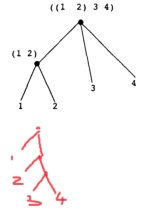``````(define c (list 1 (list 2 (list 3 (list 4 (list 5 (list 6 7)))))))
(printf "~s\n" ;for 7
(car (cdr (car (cdr (car (cdr (car (cdr (car (cdr (car (cdr c)))))))))))))
(define c2 '(1 (2 (3 (4 (5 (6 7)))))))
(printf "~s\n" ;for 7
(car (cdr (car (cdr (car (cdr (car (cdr (car (cdr (car (cdr c2)))))))))))))
(define c3 (cons 1 (cons 2 (cons 3 (cons 4 (cons 5 (cons 6 7)))))))
(printf "~s\n" (cdr (cdr (cdr (cdr (cdr (cdr c3)))))))
``````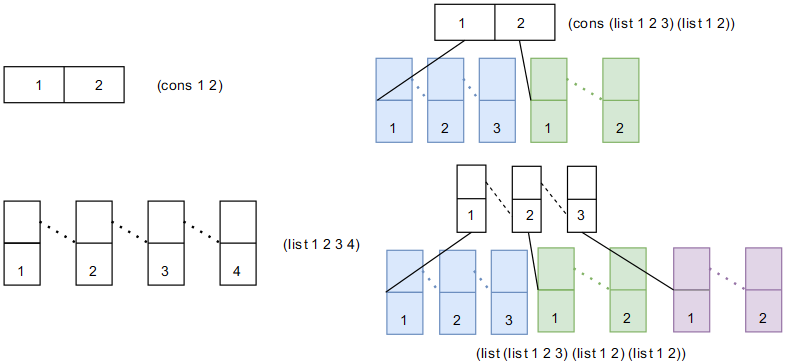``````(define (count-leaves x) ;count leaves for tree
(cond ((null? x) 0) ;end-point
((not (pair? x)) 1) ;leaf
(else (+ (count-leaves (car x)) ;node-head
(count-leaves (cdr x)))))) ;node-tail
(printf "~d\n" (count-leaves (list (list 1 2) 3 4)))
``````

``````(define (deep-reverse items)
(cond ((null? items) '())
((not (pair? items)) items) ;for list, cadr to get rest
(else (reverse (list (deep-reverse (car items))
(printf "~s\n" (deep-reverse '((1 2) (3 4))))
``````

``````(define (fringe tree)
(cond ((null? tree) '())
((not (pair? tree)) (list tree))
(else (append (fringe (car tree)) (fringe (cadr tree))))))
(define x '((1 2) (3 4)))
(printf "~s ~s\n" (fringe x) (fringe (list x x)))
``````

``````#| (define (make-mobile left right) (list left right))
(define (make-branch length structure) (list length structure))
(define (left-branch mobile) (car mobile))
(define (branch-length branch) (car branch))
(define (branch-structure branch) (cadr branch)) |#
(define (make-mobile left right) (cons left right))
(define (make-branch length structure) (cons length structure))
(define (left-branch mobile) (car mobile))
(define (right-branch mobile) (cdr mobile))
(define (branch-length branch) (car branch))
(define (branch-structure branch) (cdr branch))
(define (total-weight mobile)
(define (bw branch)
;分支重量等于：如果分支挂着重物，则是重物重量
;如果分支作为一个活动体，则返回子活动体重量
(if (pair? (branch-structure branch))
(aw (branch-structure branch))
(branch-structure branch)))
(define (aw mobile)
;活动体重量等于左边分支重量 + 右边分支重量
(+ (bw (left-branch mobile)) (bw (right-branch mobile))))
(aw mobile))
(define (is-balance mobile)
;对于节点，平衡意味着左侧分支和右侧分支力矩相等且分支均平衡
(define (mobile-balance? mobile)
(let ((left (left-branch mobile))
(right (right-branch mobile)))
(and (same-weight? left right)
(branch-balance? left)
(branch-balance? right))))
(define (branch-balance? branch)
;对于分支，如果仅挂重物，则平衡，反之判断挂载节点是否平衡
(let ((bs (branch-structure branch)))
(if (pair? bs) (mobile-balance? bs) #t)))
(define (same-weight? left right)
;左右两侧力矩是否相等：长度 * 重量，重量递归计算
(define (branch-weight branch)
(if (pair? (branch-structure branch))
(total-weight (branch-structure branch))
(branch-structure branch)))
(define (total-weight mobile)
(+ (branch-weight (left-branch mobile))
(branch-weight (right-branch mobile))))
(= (* (branch-weight left) (branch-length left))
(* (branch-weight right) (branch-length right))))
(mobile-balance? mobile))
(define m1 (make-mobile (make-branch 10 100)
(make-branch 20 200)))
(define balance-mobile (make-mobile (make-branch 10 10)
(make-branch 10 10)))
(define unbalance-mobile (make-mobile (make-branch 10 balance-mobile)
(make-branch 10 200)))
(printf "mobile weight:~d\n" (total-weight m1))
(printf "is b-m balanced? ~s\n" (is-balance balance-mobile))
(printf "is ub-m balanced? ~s\n" (is-balance unbalance-mobile))
``````

``````(define (tree-map tree action)
(cond ((null? tree) '())
((not (pair? tree)) (action tree))
(else (cons (tree-map (car tree) action)
(tree-map (cdr tree) action)))))
(define (square-tree tree)
(cond ((null? tree) '())
((not (pair? tree)) (* tree tree))
(else (cons (square-tree (car tree)) (square-tree (cdr tree))))))
(define (square-tree-2 tree) (tree-map tree (lambda (x) (* x x))))
(printf "~s ~s\n"
(square-tree '(1 (2 (3 4) 5) (6 7)))
(square-tree-2 '(1 (2 (3 4) 5) (6 7))))
``````

### (序列)作为一种约定的界面

``````(define (filter pre seq)
(cond ((null? seq) '())
((pre (car seq))
(cons (car seq) (filter pre (cdr seq))))
(else (filter pre (cdr seq)))))
(define (fold op init seq)
(if (null? seq) init (op (car seq) (fold op init (cdr seq)))))
``````

fold 能够拓宽序列这一数据抽象的应用范围，下面是一些例子，比如计算一棵树的奇数的平方和 sum-odd-squares，计算从 0 到 n 的计数斐波契些数 even-fibs，计算从 0 到 n 的斐波那契数平方，计算一个序列偶数的平方的乘积，计算公司程序员的最高收入。说实话，如果没有 fold 这个过程抽象，这些应用的实现过程将看起来千差万别，凭谁第一眼看上去也不会觉得能有一个共同的模板出来。fold 的本质在于：它提供了一种对序列每个元素按照某个过程进行整合的抽象，这种抽象对序列和整合没有任何假设，只要求序列可逐个迭代提供值，直到结束，整合可操作某个内存位置的变量，仅此而已。

``````(define (demo-of-data-abstraction)
(define odd? (lambda (x) (= (remainder x 2) 0)))
(define even? (lambda (x) (not (= (remainder x 2) 0))))
(define square (lambda (x) (* x x)))
(define (enumerate-interval low high)
(if (> low high) '()
(cons low (enumerate-interval (+ low 1) high))))
(define (fib n)
(define (iter a b count)
(if (= count 0) b (iter (+ a b) a (- count 1))))
(iter 1 0 n))

(define (sum-odd-squares tree)
(fold + 0 (map square (filter odd? (fringe tree)))))

(define (even-fibs n)
(fold cons '() (filter even? (map fib (enumerate-interval 0 n)))))

(define (list-fib-squares n)
(fold cons '() (map square (map fib (enumerate-interval 0 n)))))

(define (product-of-square-of-odd-elements seq)
(fold * 1 (map square (filter odd? seq))))

(define (salary-of-highest-paid-programmer people-list)
(define programmer? ???)
(define salary ???)
(fold max 0 (map salary (filter programmer? people-list)))) '())
``````

fold 充分展现出了抽象之美，可以使用它来实现 map, append, length 这些过程，可以看到，map 无非是 fold 对每个遍历元素应用外部操作并进行 cons 重新整合，append 无非是 fold 对于一个序列进行遍历并通过 cons 整合到另一个序列，length 无非是对一个序列进行遍历并对 0 开始累加。

``````(define (map p seq)
(fold (lambda (x y) (cons (p x) y)) '() seq))
(define (append seq1 seq2)
(fold cons seq2 seq1))
(define (length seq)
(fold (lambda (x y) (+ y 1)) 0 seq))
``````

fold 的功能不仅如此，它还可以直接应用到多个问题解决上，比如用 horner 方法计算多项式函数结果，基于 length 来统计树的叶子个数。

``````(define (horner-eval x coeff-seq)
;计算多项式函数结果，coeff-seq 为从低到高项的因子
(fold (lambda (this-coeff higher-terms)
(+ this-coeff (* higher-terms x))) 0 coeff-seq))
(printf "~s\n" (horner-eval 2 '(1 3 0 5 0 1)))
(define (count-leaves tree)
(define len (lambda (x) (if (not (pair? x)) 1 (length x))))
(fold + 0 (map len tree)))
(printf "~s\n" (count-leaves '((1 2) 3 4)))
``````

``````(define (fold-n op init seqs)
;seqs 是一个嵌套的序列，其返回一个和 seqs 等长的序列，其中每一项都是
;seqs 每个子序列特定位置的 fold 结果
(if (null? (car seqs)) '()
(cons (fold op init (map car seqs))
(fold-n op init (map cdr seqs)))))
(printf "~s\n" (fold-n + 0 '((1 2 3)(4 5 6)(7 8 9)(10 11 12))))
``````

``````;假设 ((1 2 3 4)(4 5 6 6)(6 7 8 9)) 是矩阵每行/列的表示，那么通过 map, fold 以及
;fold-n 可建立 dot-product, matrix-vector, transpose, matrix-matrix 的抽象
(define (dot-product v w)
(fold + 0 (map * v w)))
(define (matrix-*-vector m v)
(map (lambda (items) (dot-product items v)) m))
(define (transpose mat)
(fold-n cons '() mat))
(define (matrix-*-matrix m n)
(let ((n-cols (transpose n)))
(map (lambda (m-col) (matrix-*-vector n-cols m-col)) m)))
``````

``````(define (fold-left op init seq)
(define (iter result rest)
(if (null? rest) result
(iter (op result (car rest)) (cdr rest))))
(iter init seq))
(define (fold-right op init seq)
(if (null? seq) init
(op (car seq) (fold-right op init (cdr seq)))))
``````

``````(define (reverse-by-left seq)
(fold-left (lambda (ele acc) (cons acc ele)) '() seq))
(define (reverse-by-right seq)
(fold-right (lambda (ele acc) (append acc (list ele))) '() seq))
(printf "~s\n" (reverse-by-left '(1 2 3 4 5)))
(printf "~s\n" (reverse-by-right '(1 2 3 4 5)))
``````

``````(define (flatmap proc seq) (fold append '() (map proc seq)))
(define (prime-sum-pairs n)
(map (lambda (pair)
(filter (lambda (pair)
(prime? (+ (car pair) (cadr pair))))
(flatmap (lambda (i)
(map (lambda (j) (list i j))
(enumerate-interval 1 (- i 1))))
(enumerate-interval 1 n)))))
(printf "~s\n" (prime-sum-pairs 10))
``````

prime-sum-pair 的另一种实现方式是先生成更精简的数对（因为 1 + 3 和 3 + 1 在上述过程中是重复的，内层循环的 j 不必大于 i 即可）：unique-pairs 实现了这一过程，prime-sum-pairs 则基于此过程抽象进行了素数过滤和展示。

``````(define (unique-pairs n)
(flatmap (lambda (i) (map (lambda (j) (list i j))
(enumerate-interval 1 (- i 1))))
(enumerate-interval 1 n)))
(define (prime-sum-pairs n)
(map (lambda (pair) (list (car pair)
(filter (lambda (pair)
(prime? (+ (car pair) (cadr pair))))
(unique-pairs n))))
``````

``````(define (permutations s)
;生成集合 s 的所有可能排列
;去除某个元素后的集合的所有可能排列加上当前元素, 然后整合起来
(define (remove x s) (filter (lambda (e) (not (= e x))) s))
(if (null? s) (list '())
(flatmap (lambda (x)
(map (lambda (p) (cons x p))
(permutations (remove x s))))
s)))
(printf "~s\n" (permutations '(1 2 3)))
``````

``````(define (sum-of-3-number-smaller-than-n-equal-s n s)
(filter (lambda (tuple)
(let ((a (car tuple))
(and (not (= a b))
(not (= b c))
(not (= a c))
(= (+ a b c) s))))
(flatmap (lambda (a)
(flatmap (lambda (b)
(map (lambda (c) (list a b c))
(enumerate-interval 1 n)))
(enumerate-interval 1 n)))
(enumerate-interval 1 n))))
(printf "SUM:\n~s\n" (sum-of-3-number-smaller-than-n-equal-s 10 15))
``````

``````(define (queens board-size)
;计算 k 列 r 行是否可行，如果可以返回 #t 不可以返回 #f
(define (is-ok now-queen-col rest-of-queens)
(if (null? rest-of-queens) #t
(and (let ((now-queen-row (car rest-of-queens)))
(and (not (= new-row ;不能在同行
now-queen-row))
(not (= new-row ;不能在对角线
(+ (- k now-queen-col) now-queen-row)))))
(is-ok (- now-queen-col 1) (cdr rest-of-queens)))))
;(printf "for row ~s in col ~s, exist: ~s\n" new-row k rest-of-queens)
(cond ((null? rest-of-queens) (list new-row))
((is-ok (- k 1) rest-of-queens)
(append (list new-row) rest-of-queens))
(else (list -1 rest-of-queens))))
(define (safe? k positions) ;新皇后是否安全
(if (null? positions) #t (not (= (car positions) -1))))
(define (queen-cols k) ;棋盘在前 k 列放皇后的布局
(if (= k 0)
(list '())
(filter (lambda (positions) (safe? k positions))
(flatmap (lambda (rest-of-queens)
;所有旧皇后的摆放方式
(map (lambda (new-row)
;对于新列的每一行, 计算新皇后可能的位置
(enumerate-interval 1 board-size)))
(queen-cols (- k 1))))))
(queen-cols board-size))
(printf "~s\n" (queens 8))
``````

### 实例：易理解的数据屏障和深刻的过程抽象 —— 打造恰好的分层

``````;基础过程：图画右边镜像
(define (beside painter) ???)
;基础过程：图画下面镜像
(define (below painter) ???)
;基础过程：垂直翻转
(define (flip-vert painter) ???)
;基础过程：水平翻转
(define (flip-horiz painter) ???)
``````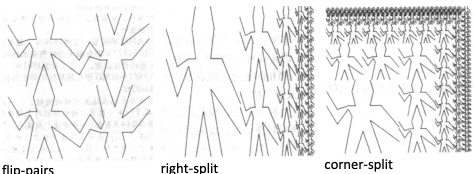``````;组合过程：垂直翻转并镜像然后上下加倍
(define (flip-pairs painter)
(let ((painter2 (beside painter (flip-vert painter))))
(below painter2 painter2)))
;组合模式：右侧分裂
(define (right-split painter n)
(if (= n 0) painter
(let ((smaller (right-split painter (- n 1))))
(beside painter (below smaller smaller)))))
;组合模式：顶部分裂
(define (up-split painter n)
(if (= n 0) painter
(let ((smaller (up-split painter (- n 1))))
(below painter (beside smaller smaller)))))
;高级组合模式：右侧分裂，顶部分裂，对角缩小镜像
(define (corner-split painter n)
(if (= n 0) painter
(let ((up (up-split painter (- n 1)))
(right (right-split painter (- n 1))))
(let ((top-left (beside up up))
(bottom-right (below right right))
(corner (corner-split painter (- n 1))))
(beside (below painter top-left)
(below bottom-right corner))))))
;高级组合模式：将上述 corner-split 水平翻转并和原始左右堆砌，
;组成的新图案垂直翻转并和原始上下堆砌
(define (square-limit painter n)
(let ((quarter (corner-split painter n)))
(let ((half (beside (flip-horiz quarter) quarter)))
(below (flip-vert half) half))))
``````

``````;过程抽象：将四个图形上下左右放置
(define (square-of-four tl tr bl br)
(lambda (painter)
(let ((top (beside (tl painter) (tr painter)))
(bottom (beside (bl painter) (br painter))))
(below bottom top))))
;基于高阶抽象重写的 flipped-pairs
(define (flipped-pairs-2 painter)
(define identity (lambda (x) x))
(let ((c4 (square-of-four
identity flip-vert identity flip-vert)))
(c4 painter)))
;基于高阶抽象重写的 square-limit
(define (square-limit-2 painter n)
(define identity (lambda (x) x))
(define rotate180 (lambda (x) (flip-horiz (flip-vert x))))
(let ((c4 (square-of-four flip-horiz identity
rotate180 flip-vert)))
(c4 (corner-split painter n))))
;同样的，可以对 up-split 和 bottom-split 进行过程抽象
(define (split action1 action2)
(define (inner painter n)
(if (= n 0) painter
(let ((smaller (inner painter (- n 1))))
(action1 painter (action2 smaller smaller)))))
(lambda (painter n) (inner painter n)))
(define right-split-2 (split beside below))
(define up-split-2 (split below beside))
``````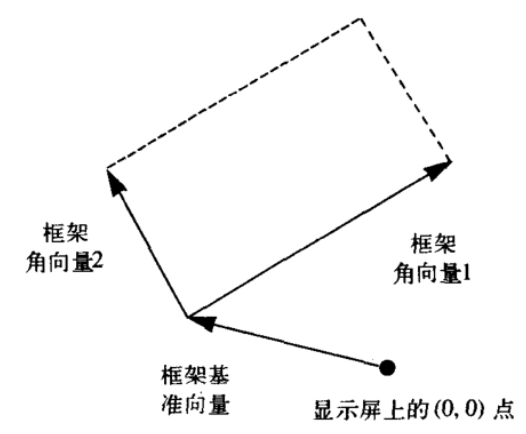``````;构建数据抽象：框架数据抽象由一个基准向量和两个角向量构成
; (define (make-frame o e1 e2) (list o e1 e2))
; (define (origin-frame f) (car f))
; (define (edge1-frame f) (cadr f))
; (define (edge2-frame f) (cadr (cdr f)))
(define (make-frame o e1 e2) (cons o (cons e1 e2)))
(define (origin-frame f) (car f))
(define (edge2-frame f) (cdr (cdr f)))
;向量数据抽象及向量的加减缩放运算
(define (make-vect x y) (cons x y))
(define (xcor-vect v) (car v))
(define (ycor-vect v) (cdr v))
(make-vect (+ (xcor-vect v1) (xcor-vect v2))
(+ (ycor-vect v1) (ycor-vect v2))))
(define (sub-vect v1 v2)
(make-vect (- (xcor-vect v1) (xcor-vect v2))
(- (ycor-vect v1) (ycor-vect v2))))
(define (scale-vect v s)
(make-vect (* s (xcor-vect v)) (* s (ycor-vect v))))
;为了将某个向量 v 映射到框架 frame，通过 frame-coord-map 进行
;v=(x,y) 到 O + xE1 + yE2 的映射
(define (frame-coord-map frame)
(lambda (v)
(origin-frame frame)
(edge1-frame frame))
(scale-vect (ycor-vect v)
(edge2-frame frame))))))
``````

``````;假设操作系统实现了 draw-line
(define (draw-line v1 v2) ???)
;基于向量建立新的数据抽象线段
(define (make-segment v1 v2) (cons v1 v2))
(define (start-segment s) (car s))
(define (end-segment s) (cdr s))
``````

``````;画家的一个定义如下，其画 segment-list 笔
;其接受一个 frame，然后对每一笔 segment 先进行坐标映射，
;然后进行绘制。画家是一个很好的“数据”抽象屏障,尽管这里的数据
;实际是使用调用系统 draw-line 的过程表示的
(define (segments->painter segment-list)
(lambda (frame)
(for-each
((lambda (segment)
(draw-line
((frame-coord-map frame) (start-segment segment))
((frame-coord-map frame) (end-segment segment))))
segment-list)))
``````

``````(define border-painter
(segments->painter
(list
(make-segment (make-vect 0 0) (make-vect 0 1))
(make-segment (make-vect 0 1) (make-vect 1 1))
(make-segment (make-vect 1 1) (make-vect 1 0))
(make-segment (make-vect 1 0) (make-vect 0 0)))))
(define x-painter
(segments->painter
(list
(make-segment (make-vect 0 0) (make-vect 1 1))
(make-segment (make-vect 0 1) (make-vect 1 0)))))
(define lozenge-painter
(segments->painter
(list
(make-segment (make-vect 0 0.5) (make-vect 0.5 1))
(make-segment (make-vect 0.5 1) (make-vect 1 0.5))
(make-segment (make-vect 1 0.5) (make-vect 0.5 0))
(make-segment (make-vect 0.5 0) (make-vect 0 0.5)))))
(define wave-painter
(segments->painter
(list
(make-segment (make-vect 0 0.8) (make-vect 0.2 0.5))
(make-segment (make-vect 0.2 0.5) (make-vect 0.3 0.6))
(make-segment (make-vect 0.3 0.6) (make-vect 0.2 0.8))
(make-segment (make-vect 0.2 0.8) (make-vect 0.3 1.0))
(make-segment (make-vect 0.7 1.0) (make-vect 0.8 0.8))
(make-segment (make-vect 0.8 0.8) (make-vect 0.7 0.6))
(make-segment (make-vect 0.7 0.6) (make-vect 0.8 0.6))
(make-segment (make-vect 0.8 0.6) (make-vect 1.0 0.3))
(make-segment (make-vect 0.0 0.5) (make-vect 0.2 0.3))
(make-segment (make-vect 0.2 0.3) (make-vect 0.3 0.5))
(make-segment (make-vect 0.3 0.5) (make-vect 0.2 0.0))
(make-segment (make-vect 0.3 0.0) (make-vect 0.5 0.3))
(make-segment (make-vect 0.5 0.0) (make-vect 0.7 0.0))
(make-segment (make-vect 0.8 0.0) (make-vect 0.7 0.5))
(make-segment (make-vect 0.7 0.5) (make-vect 1.0 0.2)))))
``````

``````(define (transform-painter painter origin c1 c2)
(lambda (frame)
(let ((m (frame-coord-map frame)))
(let ((new-origin (m origin)))
(painter
(make-frame new-origin
(sub-vect (m c1) new-origin)
(sub-vect (m c2) new-origin)))))))

(define (flip-vert painter)
(transform-painter painter (make-vect 0.0 1.0)
(make-vect 1.0 1.0) (make-vect 0.0 0.0)))
(define (shrink-to-upper-right painter)
(transform-painter painter (make-vect 0.5 0.5)
(make-vect 1.0 0.5) (make-vect 0.5 1.0)))
(define (rotate90 painter)
(transform-painter painter (make-vect 1.0 0.0)
(make-vect 1.0 1.0) (make-vect 0.0 0.0)))
(define (squash-inwards painter)
(transform-painter painter (make-vect 0.0 0.0)
(make-vect 0.65 0.35) (make-vect 0.35 0.65)))
``````

``````(define (beside painter1 painter2)
(let ((split-point (make-vect 0.5 0.0)))
(let ((paint-left
(transform-painter painter1
(make-vect 0.0 0.0)
split-point
(make-vect 0.0 1.0)))
(paint-right
(transform-painter painter2
split-point
(make-vect 1.0 0.0)
(make-vect 0.5 1.0))))
(lambda (frame)
(paint-left frame)
(paint-right frame)))))
(define (flip-horiz painter)
(lambda (frame) (transform-painter
painter
(make-vect 1.0 0.0)
(make-vect 0.0 0.0)
(make-vect 1.0 1.0))))
(define (rotate180 painter)
(lambda (frame) (transform-painter
painter
(make-vect 1.0 1.0)
(make-vect 0.0 1.0)
(make-vect 1.0 0.0))))
(define (rotate270 painter)
(lambda (frame) (transform-painter
painter
(make-vect 1.0 0.0)
(make-vect 1.0 1.0)
(make-vect 0.0 0.0))))
(define (below painter1 painter2)
(let ((split-point (make-vect 0.0 0.5)))
(let ((paint-top
(transform-painter painter2
split-point
(make-vect 1.0 0.5)
(make-vect 0.0 1.0)))
(paint-bottom
(transform-painter painter1
(make-vect 0.0 0.0)
(make-vect 1.0 0.0)
split-point)))
(lambda (frame)
(paint-top frame)
(paint-bottom frame)))))
(define (below2 painter1 painter2)
(flip-horiz (rotate90
(beside (rotate270 painter1)
(rotate270 painter2)))))
``````

## 符号数据

``````(define (memq item x)
(cond ((null? x) #f)
((eq? item (car x)) x)
(else (memq item (cdr x)))))
(define (equal? x y)
(cond ((and (symbol? x) (symbol? y)) (eq? x y))
((and (number? x) (number? y)) (= x y))
((and (list? x) (list? y))
(cond ((and (null? x) (null? y)) #t)
((or (null? x) (null? y)) #f)
((equal? (car x) (car y)) (equal? (cdr x) (cdr y)))
(else #f)))
(else #f)))
(printf "~s\n~s\n" (equal? '(this is a list) '(this is a list))
(equal? '(this is a list) '(this (is a) list)))
``````

### 实例：符号求导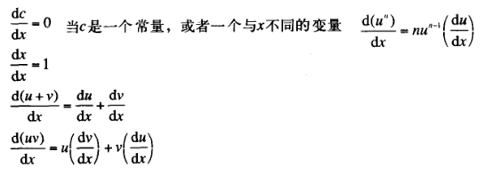Scheme 通过 symbol? 过程来判断一个表达式是否是一个符号，配合 eq? 可以实现符号等同行检测。

``````(define (variable? e) (symbol? e)) ;e是变量吗
(define (same-variable? v1 v2)
(and (variable? v1) (variable? v2) (eq? v1 v2))) ;v1 和 v2 是同一变量吗
(define (=number? exp num) (and (number? exp) (= exp num)))
(define (single-operand? x) (null? (cdr x)))
``````

``````(define (sum? e)  (and (pair? e) (eq? (car e) '+))) ;e是和式吗
;加法运算基本定义（仅支持两个数）
(define (augend-simple e) (caddr e)) ;e的加数
(define (make-sum-simple a1 a2)
(cond ((=number? a1 0) a2)
((=number? a2 0) a1)
((and (number? a1) (number? a2)) (+ a1 a2))
(else (list '+ a1 a2)))) ;构造a1 和 a2 的和式
;加法运算高级定义（支持多个加数）
(define (augend e)
(let ((tail (cddr e)))
(if (single-operand? tail)
(car tail)
(apply make-sum tail)))) ;e的加数
(define (make-sum a1 . a2)
(if (single-operand? a2)
(make-sum-simple a1 (car a2))
(cons '+ (cons a1 a2))))
``````

``````(define (product? e)  (and (pair? e) (eq? (car e) '*))) ;e是乘式吗
(define (multiplier e) (cadr e)) ;e的被乘数
;乘法运算基本定义（仅支持两个数）
(define (multiplicand-simple e) (caddr e)) ;e的乘数
(define (make-product-simple m1 m2)
(cond ((or (=number? m1 0) (=number? m2 0)) 0)
((=number? m1 1) m2)
((=number? m2 1) m1)
((and (number? m1) (number? m2)) (* m1 m2))
(else (list '* m1 m2)))) ;构造 m1 和 m2 的乘式
;乘法运算高级定义（支持多个乘数）
(define (multiplicand e)
(let ((tail (cddr e)))
(if (single-operand? tail)
(car tail)
(apply make-product tail))))
(define (make-product m1 . m2)
(if (single-operand? m2)
(make-product-simple m1 (car m2))
(cons '* (cons m1 m2))))
``````

``````;阶乘运算定义
(define (exponentiation? e) (and (pair? e) (eq? (car e) '**)))
(define (make-exponentiation e1 e2)
(cond ((=number? e2 0) 1)
((=number? e2 1) e1)
(else (list '** e1 e2))))
``````

``````;求导过程实现
(define (deriv exp var)
(cond ((number? exp) 0)
((variable? exp)
(if (same-variable? exp var) 1 0))
((sum? exp)
(deriv (augend exp) var)))
((product? exp)
(make-sum
(make-product (multiplier exp)
(deriv (multiplicand exp) var))
(make-product (deriv (multiplier exp) var)
(multiplicand exp))))
((exponentiation? exp)
(make-product
(make-product ((exponent exp)
(make-exponentiation (base exp)
(- (exponent exp) 1))))
(deriv (base exp) var)))
(else (error "unknow expression type -- DERIV" exp))))
(printf "~s\n" (deriv '(+ x 3) 'x))
(printf "~s\n" (deriv '(* x y) 'x))
(printf "~s\n" (deriv '(* (* x y) (+ x 3)) 'x))
(printf "~s\n" (deriv '(* x y (+ x 3)) 'x)))
``````

``````;基本过程定义
(define (variable? e) (symbol? e)) ;e是变量吗
(define (same-variable? v1 v2)
(and (variable? v1) (variable? v2) (eq? v1 v2))) ;v1 和 v2 是同一变量吗
(define (=number? exp num) (and (number? exp) (= exp num)))
(define (single-operand? x) (null? (cdr x))) ;x + (3 + 4)
;加法运算基本定义
(define (sum? e)  (and (pair? e) (eq? (cadr e) '+) (not (null? (cddr e))))) ;e是和式吗
(define (addend e) (car e)) ;e的被加数
;加法运算基本定义（仅支持两个数）
(define (augend e) (caddr e)) ;e的加数
(define (make-sum a1 a2)
(cond ((=number? a1 0) a2)
((=number? a2 0) a1)
((and (number? a1) (number? a2)) (+ a1 a2))
(else (list a1 '+ a2)))) ;构造a1 和 a2 的和式
; ;加法运算高级定义（支持多个加数）
; (define (augend e) ;(a + b + c + d)
;     (let ((tail (cddr e)))
;         (if (single-operand? tail)
;             (car tail) ;(b) -> b or (b + c + d) -> ? 错误，这里有乘法优先级问题
;这里充分体现了前缀表达式在传递多个参数上的优势，根本无需担心运算符改变问题
;             (cond ((sum? tail) (apply make-sum tail))
;                   ((product? tail) (apply make-product tail)))))) ;e的加数
;乘法运算基本定义
(define (product? e)  (and (pair? e) (eq? (cadr e) '*) (not (null? (cddr e))))) ;e是乘式吗
(define (multiplier e) (car e)) ;e的被乘数
;乘法运算基本定义（仅支持两个数）
(define (multiplicand e) (caddr e)) ;e的乘数
(define (make-product m1 m2)
(cond ((or (=number? m1 0) (=number? m2 0)) 0)
((=number? m1 1) m2)
((=number? m2 1) m1)
((and (number? m1) (number? m2)) (* m1 m2))
(else (list m1 '* m2)))) ;构造 m1 和 m2 的乘式
;求导过程实现
(define (deriv exp var)
(cond ((number? exp) 0)
((variable? exp)
(if (same-variable? exp var) 1 0))
((sum? exp)
(deriv (augend exp) var)))
((product? exp)
(make-sum
(make-product (multiplier exp)
(deriv (multiplicand exp) var))
(make-product (deriv (multiplier exp) var)
(multiplicand exp))))
(else (error "unknow expression type -- DERIV" exp)))
(printf "~s\n" (deriv '(x + 3) 'x))
(printf "~s\n" (deriv '(x * y) 'x))
(printf "~s\n" (deriv '((x * y) * (x + 3)) 'x)))
``````

### 实例：集合的表示

``````; (define (element-of-set? e u) ???)
; (define (adjoin-set s u) ???)
; (define (union-set u1 u2) ???)
; (define (intersection-set u1 u2) ???)
``````

``````;不允许重复的未排序数据抽象
(define (element-of-set? e u)
(cond ((null? u) #f)
((equal? e (car u)) #t)
(else (element-of-set? e (cdr u)))))
(if (element-of-set? u s) u (cons s u)))
(define (union-set u1 u2)
(fold (lambda (ele-of-u2 u1-now)
(if (element-of-set? ele-of-u2 u1-now)
u1-now
(cons ele-of-u2 u1-now))) u1 u2))
(define (intersection-set u1 u2)
(cond ((or (null? u1) (null? u2)) '())
((element-of-set? (car u1) u2)
(cons (car u1) (intersection-set (cdr u1) u2)))
(else (intersection-set (cdr u1) u2))))
(printf "~s ~s ~s\n" (element-of-set? 1 '(1 2 3))
(element-of-set? 1 '(2 3))
(element-of-set? 1 '()))
(printf "~s ~s\n" (union-set '(1 2 3) '(2 3 4))
(intersection-set '(1 2 3) '(2 3 4))))
``````

``````;允许重复的未排序数据抽象
(define (element-of-set? e u)
(cond ((null? u) #f)
((equal? e (car u)) #t)
(else (element-of-set? e (cdr u)))))
(define (adjoin-set s u) (cons s u))
(define (union-set u1 u2)
(fold (lambda (ele-of-u2 u1-now)
(if (element-of-set? ele-of-u2 u1-now)
u1-now
(cons ele-of-u2 u1-now))) u1 u2))
(define (intersection-set u1 u2)
(fold (lambda (ele-of-u2 res-now)
(if (and (element-of-set? ele-of-u2 u1)
(not (element-of-set? ele-of-u2 res-now)))
(cons ele-of-u2 res-now) res-now)) '() u2))
(printf "~s ~s ~s\n" (element-of-set? 1 '(1 2 2 3))
(element-of-set? 1 '(2 3))
(element-of-set? 1 '()))
(printf "~s ~s\n" (union-set '(1 2 2 3) '(2 3 3 4))
(intersection-set '(1 2 3 3) '(2 3 4 4)))
``````

``````;排序数据抽象
(define (element-of-set? e u)
(cond ((null? u) #f)
((= e (car u)) #t)
((< e (car u)) #f)
(else (element-of-set? e (cdr u)))))
(if (null? u) (cons s '())
(let ((next (car u)))
(if (< s next) (cons s u)
(cons next (adjoin-set s (cdr u)))))))
(define (union-set u1 u2)
(cond ((null? u1) u2)
((null? u2) u1)
(else (let ((e1 (car u1)) (e2 (car u2)))
(cond ((= e1 e2) (cons e1 (union-set (cdr u1) (cdr u2))))
((> e1 e2) (cons e2 (union-set u1 (cdr u2))))
((< e1 e2) (cons e1 (union-set (cdr u1) u2))))))))
(define (intersection-set u1 u2)
(if (or (null? u1) (null? u2)) '()
(let ((e1 (car u1)) (e2 (car u2)))
(cond ((= e1 e2) (cons e1 (intersection-set (cdr u1) (cdr u2))))
((> e1 e2) (intersection-set u1 (cdr u2)))
((< e1 e2) (intersection-set (cdr u1) u2))))))
````````````;基于二叉树实现的数据抽象
(define (entry tree) (car tree))
(define (make-tree entry left right) (list entry left right))
``````

``````(define (element-of-set? x set)
(cond ((null? set) #f)
((= x (entry set)) #t)
((< x (entry set))
(element-of-set? x (left-branch set)))
((> x (entry set))
(element-of-set? x (right-branch set)))))
(cond ((null? set) (make-tree x '() '()))
((= x (entry set)) set)
((< x (entry set))
(make-tree (entry set)
(right-branch set)))
((> x (entry set))
(make-tree (entry set)
(left-branch set)
``````

``````(define (tree->list-1 tree)
(if (null? tree) '()
(append (tree->list-1 (left-branch tree))
(cons (entry tree)
(tree->list-1 (right-branch tree))))))
(define (tree->list-2 tree) ;先解决左边值，然后解决其右边的兄弟值
;这种方式会下到最小的最左边节点，依次解决底层，弹出栈，往上游走
;直到根节点，然后在右侧子树也是如此，最后被解决的是最大的最右节点，
;其没有任何的左节点，向上依次弹出栈到根
(define (copy-to-list tree result-list)
(if (null? tree) result-list
(copy-to-list (left-branch tree)
(cons (entry tree)
(copy-to-list (right-branch tree)
result-list)))))
(copy-to-list tree '()))
(define t1 (make-tree 7
(make-tree 3 (make-tree 1 '() '()) (make-tree 5 '() '()))
(make-tree 9 '() (make-tree 11 '() '()))))
(define t2 (make-tree 3
(make-tree 1 '() '())
(make-tree 7
(make-tree 5 '() '())
(make-tree 9 '() (make-tree 11 '() '())))))
(define t3 (make-tree 5
(make-tree 3 (make-tree 1 '() '()) '())
(make-tree 9 (make-tree 7 '() '()) (make-tree 11 '() '()))))
``````

``````(define (list->tree elements)
(car (partial-tree elements (length elements))))
(define (partial-tree elts n)
(if (= n 0) (cons '() elts) ;如果没有需要处理的元素，则返回空树
(let ((left-size (quotient (- n 1) 2)))
(let ((left-result (partial-tree elts left-size))) ;先处理一半
(let ((left-tree (car left-result)) ;左边的树
(non-left-elts (cdr left-result)) ;左边尚未处理的元素
(right-size (- n (+ left-size 1)))) ;剩下的右边处理（除了当前根）
(let ((this-entry (car non-left-elts)) ;当前根
(right-result (partial-tree (cdr non-left-elts) right-size))) ;后处理一半
(let ((right-tree (car right-result)) ;右边的树
(remaining-elts (cdr right-result))) ;剩余未处理元素
(cons (make-tree this-entry left-tree right-tree)
remaining-elts)))))))) ;将左树和右树构造起来，剩余元素返回
``````

``````(define (union-set t1 t2)
(define (union-sorted-set u1 u2)
(cond ((null? u1) u2)
((null? u2) u1)
(else (let ((e1 (car u1)) (e2 (car u2)))
(cond ((= e1 e2) (cons e1 (union-sorted-set (cdr u1) (cdr u2))))
((> e1 e2) (cons e2 (union-sorted-set u1 (cdr u2))))
((< e1 e2) (cons e1 (union-sorted-set (cdr u1) u2))))))))
(list->tree (union-sorted-set (tree->list-2 t1) (tree->list-2 t2))))
(define (intersection-set t1 t2)
(define (intersection-sorted-set u1 u2)
(if (or (null? u1) (null? u2)) '()
(let ((e1 (car u1)) (e2 (car u2)))
(cond ((= e1 e2) (cons e1 (intersection-sorted-set (cdr u1) (cdr u2))))
((> e1 e2) (intersection-sorted-set u1 (cdr u2)))
((< e1 e2) (intersection-sorted-set (cdr u1) u2))))))
(list->tree (intersection-sorted-set (tree->list-2 t1) (tree->list-2 t2))))
``````

``````(define (lookup given-key set-of-records)
(define (key set) ???)
(cond ((null? set-of-records) #f)
((equal? given-key (key (car set-of-records)))
(car set-of-records))
(else (lookup given-key (cdr set-of-records)))))
(define (lookup-2 given-key tree)
(define (key tree) ???)
(if (null? tree) #f
(let ((node (entry tree)))
(let ((node-key (key node)))
(cond ((= node-key given-key) node)
((< node-key given-key)
(lookup-2 given-key (right-branch tree)))
((> node-key given-key)
(lookup-2 given-key (left-branch tree))))))))
(printf " ~s\n ~s\n ~s\n" (tree->list-1 t1) (tree->list-1 t2) (tree->list-1 t3))
(printf " ~s\n ~s\n ~s\n" (tree->list-2 t1) (tree->list-2 t2) (tree->list-2 t3))
(printf "~s\n" (list->tree '(1 3 5 7 9 11)))
(printf "~s\n" (intersection-set (list->tree '(1 2 3 4 5)) (list->tree '(1 3 5 7 9))))
(printf "~s\n" (union-set (list->tree '(1 2 3 4 5)) (list->tree '(1 3 5 7 9)))))
``````

### 实例：霍夫曼编码树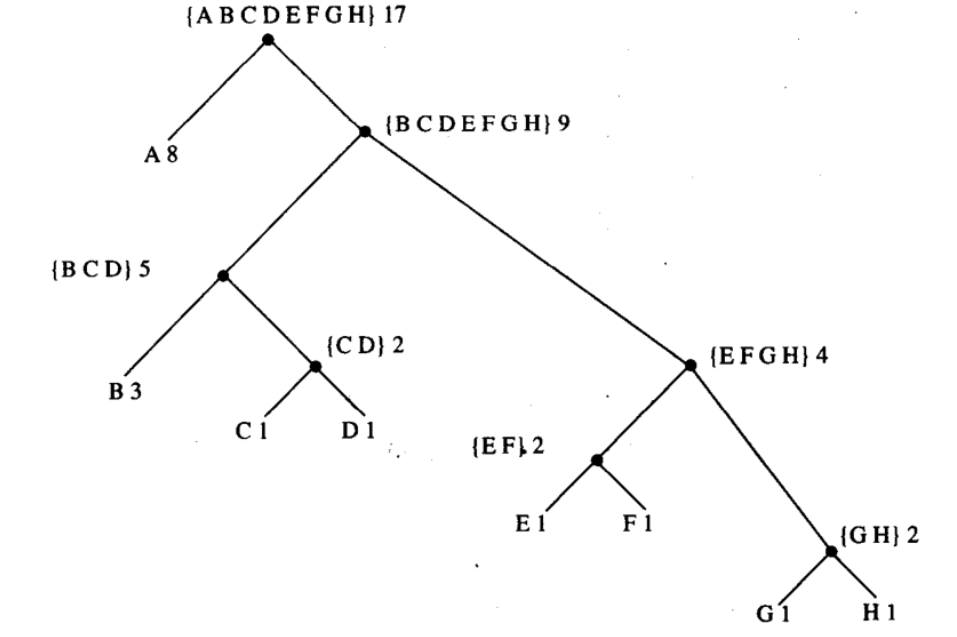``````(define (make-leaf symbol weight) ;制作字典节点
(list 'leaf symbol weight))
(define (leaf? obj)
(eq? (car obj) 'leaf))
(define (make-code-tree left right) ;制作字典
(list left right
(append (symbols left) (symbols right))
(+ (weight left) (weight right))))
(define (left-branch tree) (car tree))
(define (symbols tree)
(if (leaf? tree) (list (symbol-leaf tree))
(define (weight tree)
(if (leaf? tree) (weight-leaf tree)
``````

decode 方法用来对一串 0 和 1 进行解码，方法很简单，根据数据在 tree 中导航，直到找到 leaf 取出符号为止，然后依次将多个符号取出来返回集合。

``````(define (decode bits tree) ;根据二进制导航左右 branch 解码
(define (choose-branch bit branch)
(cond ((= bit 0) (left-branch branch))
((= bit 1) (right-branch branch))
(else (error "bad bit from choose-branch" bit))))
(define (decode-1 bits current-branch)
(if (null? bits) '()
(let ((next-branch (choose-branch (car bits) current-branch)))
(if (leaf? next-branch)
(cons (symbol-leaf next-branch)
(decode-1 (cdr bits) tree))
(decode-1 (cdr bits) next-branch)))))
(decode-1 bits tree))
``````

encode 方法用来将一串符号进行编码，对于每一个符号，都通过 tree 判断左树和右树是否包含，然后进入左树记下 0 右树记下 1，最后得到一个符号的 0 1 序列，不断如此，得到所有符号的 0 1 序列。

``````(define (encode message tree)
;对消息 message 通过 tree 字典进行编码，返回二进制序列
(define (contains items x)
(cond ((null? items) #f)
((eq? (car items) x) #t)
(else (contains (cdr items) x))))
(define (encode-symbol x tree)
(cond ((leaf? tree) '())
((contains (symbols (left-branch tree)) x)
(cons 0 (encode-symbol x (left-branch tree))))
((contains (symbols (right-branch tree)) x)
(cons 1 (encode-symbol x (right-branch tree))))
(define (encode-inner message)
(if (null? message) '()
(append (encode-symbol (car message) tree)
(encode-inner (cdr message)))))
(encode-inner message))
``````

``````(define sample-tree
(make-code-tree
(make-leaf 'A 4)
(make-code-tree
(make-leaf 'B 2)
(make-code-tree
(make-leaf 'D 1)
(make-leaf 'C 1)))))
(define codec-message (encode '(B B D C A C) sample-tree))
(define decode-message (decode codec-message sample-tree))
(printf "~s ~s\n" codec-message decode-message)
``````

``````(define (generate-huffman-tree pairs)
(define (adjoin-set x set) ;将 x 插入到有序 set 中
(cond ((null? set) (list x))
((> (weight x) (weight (car set))) (cons x set))
(else (cons (car set) (adjoin-set x (cdr set))))))
(define (make-leaf-set pairs)
;将 ((A 4) (B 2) (C 1)) 转换为排序 leaf 集合
(if (null? pairs) '()
(let ((pair (car pairs)))
(make-leaf-set (cdr pairs))))))
(define (successive-merge set)
(if (null? set) (make-leaf 'EOF -1)
(make-code-tree
(car set) (successive-merge (cdr set)))))
(successive-merge (make-leaf-set pairs)))
``````

``````(define h-tree (generate-huffman-tree '((A 4) (B 3) (C 2))))
(printf "~s\n~s\n~s\n" h-tree
(encode '(C A B) h-tree)
(decode '(1 1 0 0 1 0) h-tree))
; ((leaf A 4)
;  ((leaf B 3)
;   ((leaf C 2)
;    (leaf END -1)
;    (C END)
;    1)
;   (B C END)
;   4)
;  (A B C END)
;  8)
``````

``````(define rock-tree (generate-huffman-tree
'((A 2) (BOOM 1) (NA 16) (SHA 3) (GET 2) (YIP 9) (JOB 2) (WAH 1))))
(define codec-rock
(encode '(GET A JOB
SHA NA NA NA NA NA NA NA NA
GET A JOB
SHA NA NA NA NA NA NA NA NA
WAH YIP YIP YIP YIP YIP YIP YIP YIP YIP
SHA BOOM) rock-tree))
(printf "~s\nlength:~d\n" codec-rock (length codec-rock))
``````

## 抽象数据的多重表示

``````;如果采用直角坐标表示
;x = rcosA, y = rsinA, r = √(x*x + y*y), a = arctan(y,x)
(define (make-from-real-imag x y) (cons x y))
(define (make-from-mag-ang r a)
(cons (* r (cos a)) (* r (sin a))))
(define (real-part x) (car x))
(define (magnitude x)
(sqrt (+ (square (real-part x))
(square (imag-part x)))))
(define (angle x)
(atan (imag-part x) (real-part x)))

;如果采用极坐标形式
(define (make-from-real-imag x y)
(cons (sqrt (+ (* x x) (* y y))) (atan y x)))
(define (make-from-mag-ang r a) (cons r a))
(define (real-part x) (* (magnitude x) (cos (angle x))))
(define (imag-part x) (* (magnitude x) (sin (angle x))))
(define (magnitude x) (car x))
``````

``````(define (add-complex z1 z2)
(make-from-real-imag (+ (real-part z1) (real-part z2))
(+ (imag-part z1) (imag-part z2))))
(define (sub-complex z1 z2)
(make-from-real-imag (- (real-part z1) (real-part z2))
(- (imag-part z1) (imag-part z2))))
(define (mul-complex z1 z2)
(make-from-mag-ang (* (magnitude z1) (magnitude z2))
(+ (angle z1) (angle z2))))
(define (div-complex z1 z2)
(make-from-mag-ang (/ (magnitude z1) (magnitude z2))
(- (angle z1) (angle z2))))
``````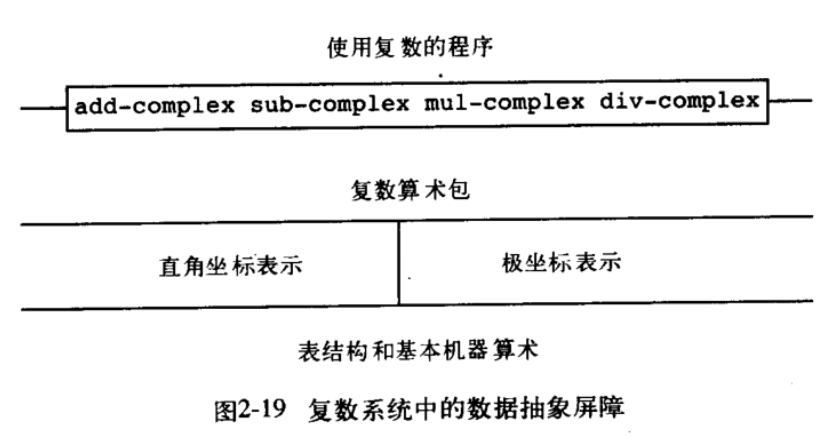``````(define (label-usage)
(define (attach-tag type content) (cons type content))
(define (type-tag datum)
(if (pair? datum) (car datum) (error "no tag find" datum)))
(define (contents datum)
(if (pair? datum) (cdr datum) (error "no content find" datum)))
``````

``````(define (rectangular? z) (eq? (type-tag z) 'rectangular))
(define (polar? z) (eq? (type-tag z) 'polar))
``````

``````(define (make-from-real-imag-r x y)
(attach-tag 'rectangular (cons x y)))
(define (make-from-mag-ang-r r a)
(attach-tag 'rectangular
(cons (* r (cos a)) (* r (sin a)))))
(define (real-part-r x) (car x))
(define (magnitude-r x)
(sqrt (+ (square (real-part-r x))
(square (imag-part-r x)))))
(define (angle-r x)
(atan (imag-part-r x) (real-part-r x)))

(define (make-from-real-imag-p x y)
(attach-tag 'polar
(cons (sqrt (+ (* x x) (* y y))) (atan y x))))
(define (make-from-mag-ang-p r a)
(attach-tag 'polar (cons r a)))
(define (real-part-p x) (* (magnitude-p x) (cos (angle-p x))))
(define (imag-part-p x) (* (magnitude-p x) (sin (angle-p x))))
(define (magnitude-p x) (car x))
``````

``````(define (real-part z)
(cond ((rectangular? z)
(real-part-r (contents z)))
((polar? z)
(real-part-p (contents z)))))
(define (imag-poart z)
(cond ((rectangular? z)
(imag-part-r (contents z)))
((polar? z)
(imag-part-p (contains z)))))
(define (magnitude z)
(cond ((rectangular? z)
(magnitude-r (contents z)))
((polar? z)
(magnitude-p (contents z)))))
(define (angle z)
(cond ((rectangular? z)
(angle-rectangular (contents z)))
((polar? z)
(angle-polar (contents z)))))
(define (make-from-real-imag x y) (make-from-real-imag-r x y))
(define (make-from-mag-ang r a) (make-from-mag-ang-p r a))
``````

``````(define operation-table (make-table))
(define get (operation-table 'lookup-proc))
(define put (operation-table 'insert-proc!))
``````

``````(define (install-r-package)
(define (make-from-real-imag x y) (cons x y))
(define (make-from-mag-ang r a)
(cons (* r (cos a)) (* r (sin a))))
(define (real-part x) (car x))
(define (magnitude x)
(sqrt (+ (square (real-part x)) (square (imag-part x)))))
(define (angle x) (atan (imag-part x) (real-part x)))

(define (tag x) (attach-tag 'rectangular x))
(put 'real-part '(rectangular) real-part)
(put 'imag-part '(rectangular) imag-part)
(put 'magnitude '(rectangular) magnitude)
(put 'angle '(rectangular) angle)
(put 'make-from-real-imag 'rectangular
(lambda (x y) (tag (make-from-real-imag x y))))
(put 'make-from-mag-ang 'rectangular
(lambda (r a) (tag (make-from-mag-ang r a)))))

(define (install-p-package)
(define (make-from-real-imag x y)
(cons (sqrt (+ (* x x) (* y y))) (atan y x)))
(define (make-from-mag-ang r a) (cons r a))
(define (real-part x) (* (magnitude x) (cos (angle x))))
(define (imag-part x) (* (magnitude x) (sin (angle x))))
(define (magnitude x) (car x))

(define (tag x) (attach-tag 'polar x))
(put 'real-part '(polar) real-part)
(put 'imag-part '(polar) imag-part)
(put 'magnitude '(polar) magnitude)
(put 'angle '(polar) angle)
(put 'make-from-real-imag 'polar
(lambda (x y) (tag (make-from-real-imag x y))))
(put 'make-from-mag-ang 'polar
(lambda (r a) (tag (make-from-mag-ang r a)))))
``````

``````(define (type-tag datum)
(if (pair? datum) (car datum) (error "no tag find" datum)))
(define (apply-generic op . args)
(let ((type-tags (map type-tag args)))
(let ((proc (get op type-tags)))
(if proc
(apply proc (map contents args))
(error "no method found." (list op type-tags))))))
(define (real-part z) (apply-generic 'real-part z))
(define (imag-part z) (apply-generic 'imag-part z))
(define (magnitude z) (apply-generic 'magnitude z))
(define (angle z) (apply-generic 'angle z))
(define (make-from-real-imag x y)
((get 'make-from-real-imag 'rectangular) x y))
(define (make-from-mag-ang r a)
((get 'make-from-mag-ang 'polar) r a))
``````

``````(define (deriv exp var)
(cond ((number? exp) 0)
((variable? exp)
(if (same-variable? exp var) 1 0))
((sum? exp)
(deriv (augend exp) var)))
((product? exp)
(make-sum
(make-product (multiplier exp)
(deriv (multiplicand exp) var))
(make-product (deriv (multiplier exp) var)
(multiplicand exp))))
(else (error "unknow expression type -- DERIV" exp))))

(define (deriv-2 exp var)
(cond ((number? exp) 0)
((variable? exp)
(if (same-variable? exp var) 1 0))
(else ((get 'deriv (operator exp)) (operands exp) var))))
(define (operator exp) (car exp))
(define (operands exp) (cdr exp))
(put 'deriv '+ (lambda (operands var)
(let ((exp (cons '+ operands)))
(deriv (augend exp) var)))))
(put 'deriv '* (lambda (operands var)
(let ((exp (cons '* operand)))
(make-sum
(make-product (multiplier exp)
(deriv (multiplicand exp) var))
(make-product (deriv (multiplier exp) var)
(multiplicand exp))))))
``````

``````(define (make-from-real-imag x y)
(define (dispatch op)
(cond ((eq? op 'real-part) x)
((eq? op 'imag-part) y)
((eq? op 'magnitude)
(sqrt (+ (square x) (square y))))
((eq? op 'angle) (atan y x))
(else (error "unknown op" op))))
dispatch)
(define (make-from-mag-ang r a)
(lambda (op)
(cond ((eq? op 'real-part) (* r (cos a)))
((eq? op 'imag-part) (* r (sin a)))
((eq? op 'magnitude) r)
((eq? op 'angle) a))))
(define (apply-generic op arg) (arg op))
``````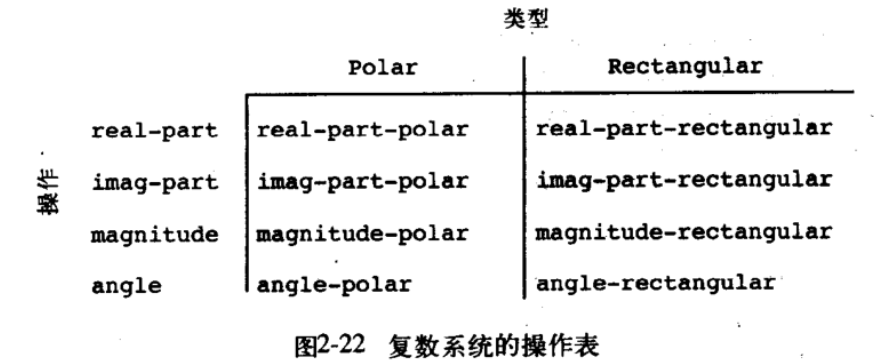## 数据抽象的层次（带有通用型操作的系统）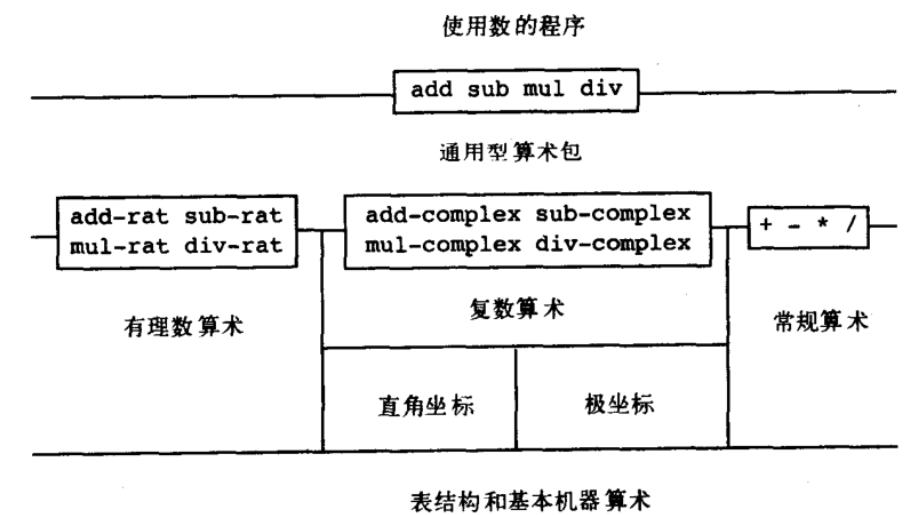``````(define (add x y) (apply-generic 'add x y))
(define (sub x y) (apply-generic 'sub x y))
(define (mul x y) (apply-generic 'mul x y))
(define (div x y) (apply-generic 'div x y))
(define (equ? x y) (append-generic 'equ x y))
(define (install-basic-number)
(define (tag x) (attach-tag 'scheme-number x))
(lambda (x y) (tag (+ x y))))
(put 'sub '(scheme-number scheme-number)
(lambda (x y) (tag (- x y))))
(put 'mul '(scheme-number scheme-number)
(lambda (x y) (tag (* x y))))
(put 'div '(scheme-number scheme-number)
(lambda (x y) (tag (/ x y))))
(put 'make 'scheme-number
(lambda (x) (tag x)))
(put 'equ? 'scheme-number
(lambda (x y) (= x y)))
'done)
(define (make-scheme-number n)
((get 'make 'scheme-number) n))
(define (install-rational)
(define (number x) (car x))
(define (denom x) (cdr x))
(define (make-rat n d) (let ((g (gcd n d)))
(cons (/ n g) (/d g))))
(make-rat (+ (* (number x) (denom x))
(* (number y) (denom y)))
(* (denom x) (denom y))))
(define (sub-rat x y)
(make-rat (- (* (number x) (denom y))
(* (number y) (denom x)))
(* (denom x) (denom y))))
(define (mul-rat x y)
(make-rat (* (number x) (number y))
(* (denom x) (denom y))))
(define (div-rat x y)
(make-rat (* (number x) (denom y))
(* (denom x) (number y))))
(define (tag x) (attach-tag 'rational x))
(lambda (x y) (tag (add-rat x y))))
(put 'sub '(rational rational)
(lambda (x y) (tag (sub-rat x y))))
(put 'mul '(rational rational)
(lambda (x y) (tag (mul-rat x y))))
(put 'div '(rational rational)
(lambda (x y) (tag (div-rat x y))))
(put 'equ? '(rational rational)
(lambda (x y)
(let ((zero (sub-rat x y)))
(and (= (number zero) 0) (= (denom zero) 0)))))
'done)
(define (make-rational n d)
((get 'make 'rational) n d))
``````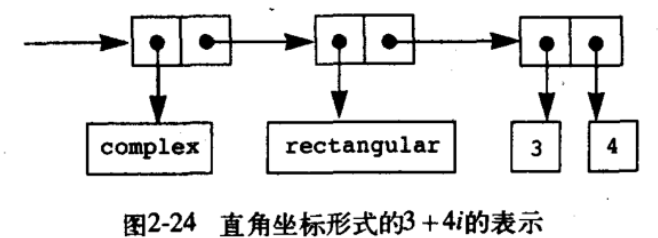``````(define (install-complex-pakcage)
(define (make-from-real-imag x y)
((get 'make-from-real-imag 'rectangular) x y))
(define (make-from-mag-ang r a)
((get 'make-from-mag-ang 'polar) r a))
(make-from-real-imag (+ (real-part z1) (real-part z2))
(+ (imag-part z1) (imag-part z2))))
(define (sub-complex z1 z2)
(make-from-real-imag (- (real-part z1) (real-part z2))
(- (imag-part z1) (imag-part z2))))
(define (mul-complex z1 z2)
(make-from-mag-ang (* (magnitude z1) (magnitude z2))
(+ (angle z1) (angle z2))))
(define (div-complex z1 z2)
(make-from-mag-ang (/ (magnitude z1) (magnitude z2))
(- (angle z1) (angle z2))))
(define (tag x) (attach-tag 'complex x))
(lambda (z1 z2) (tag (add-complex z1 z2))))
(put 'sub '(complex complex)
(lambda (z1 z2) (tag (sub-complex z1 z2))))
(put 'mul '(complex complex)
(lambda (z1 z2) (tag (mul-complex z1 z2))))
(put 'div '(complex complex)
(lambda (z1 z2) (tag (div-complex z1 z2))))
(put 'make-from-real-imag 'complex
(lambda (x y) (tag (make-from-real-imag x y))))
(put 'make-from-mag-ang 'complex
(lambda (r a) (tag (make-from-mag-ang r a))))
(put 'equ? '(complex complex)
(lambda (x y)
(let ((zero (sub-complex x y)))
(and (= (real-part zero) 0)
(= (imag-part zero) 0)))))
'done)
(define (make-complex-from-real-imag x y)
((get 'make-from-real-imag 'complex) x y))
(define (make-complex-from-mag-ang r a)
((get 'make-from-mag-ang 'complex) r a))
``````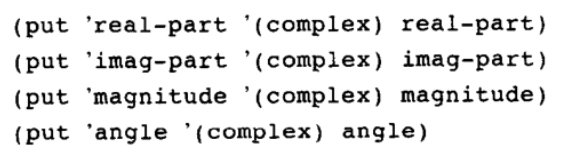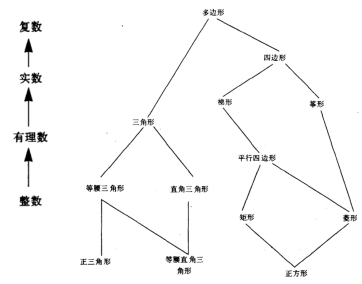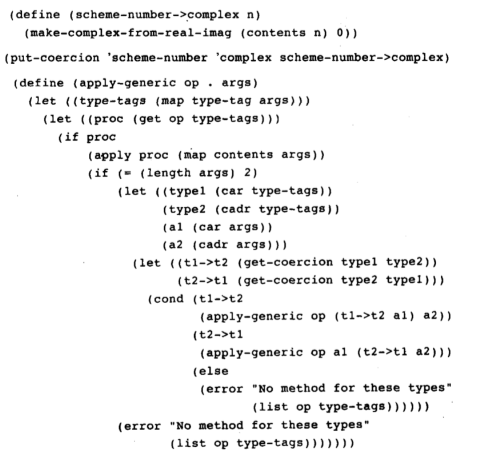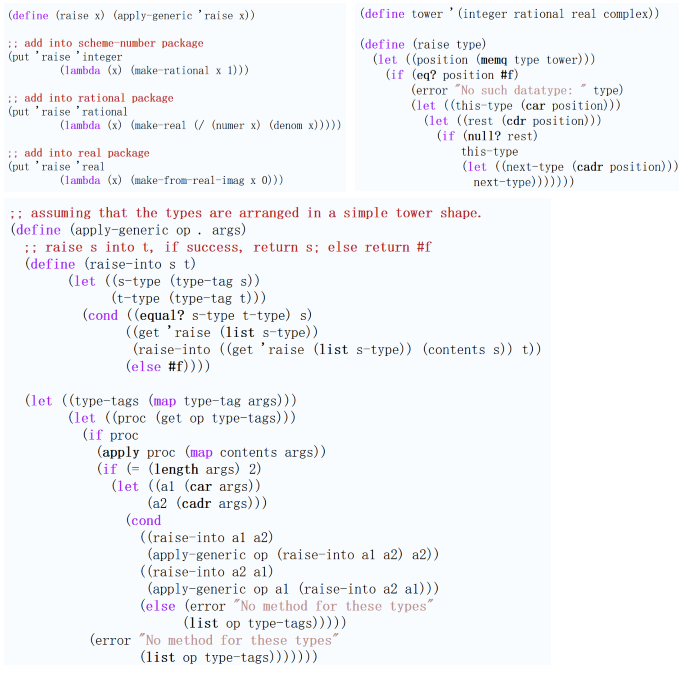## 消息传递风格 (无状态面向对象) 中的数据和过程抽象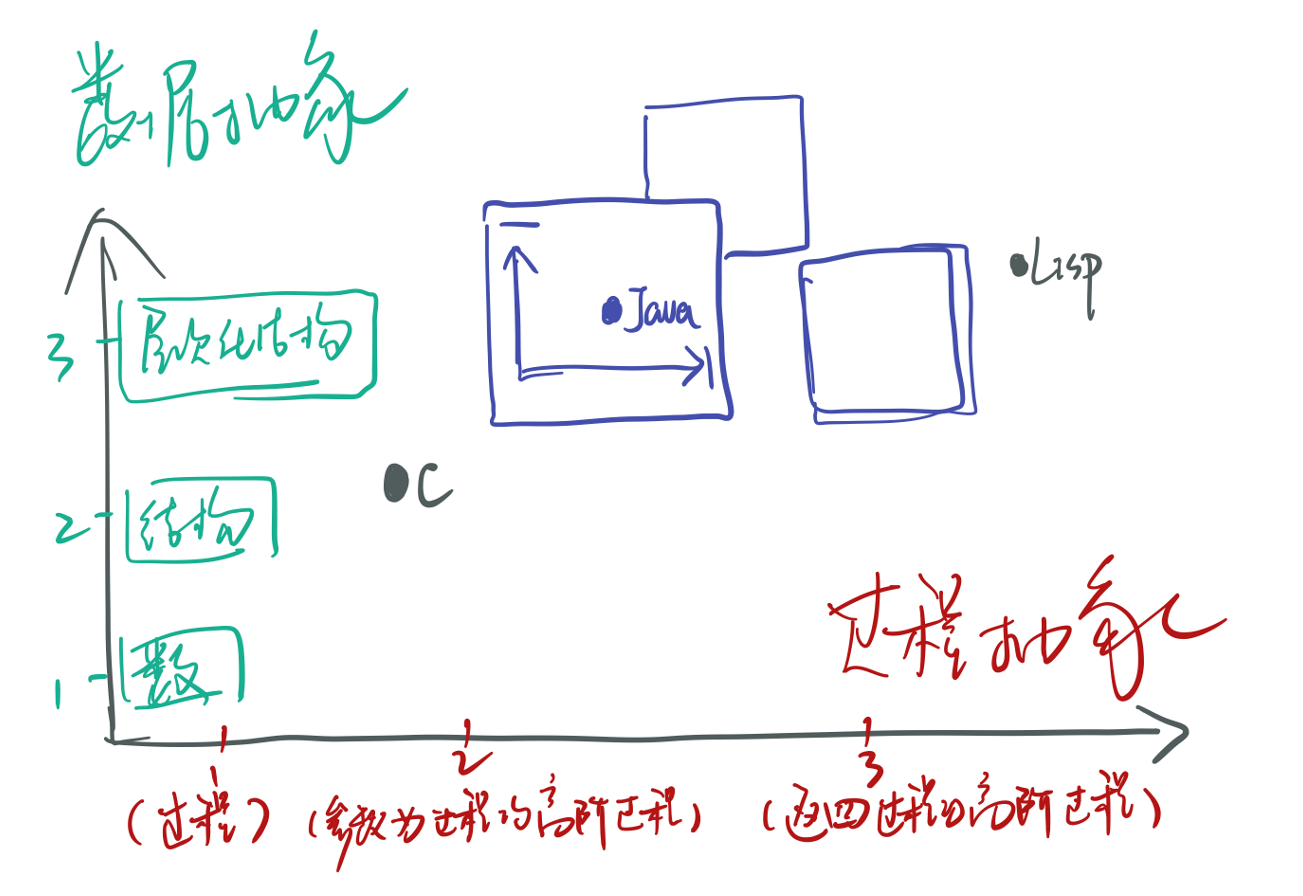Ps. 在面向对象和设计模式中被广泛提到的“面向接口编程”其实本意应该是“不要打破数据抽象的封装”，后者并不是前者反向的论述，其包含范围更广，这里数据抽象的封装包括（a）数据不同表现形式的封装以及（b）数据抽象底层实现的封装。大部分设计模式教材中提到的“面向接口”更偏向前者 —— 接口和实现类的解耦，但实际上经典的设计模式在生产中比较少见，数据的多种表现形式并不总会有，但相反，数据的基础封装却很多，而往往被忽视的则总是不要破坏数据抽象这一部分 —— 在面向对象语言中，破坏数据抽象的典型表现就是级联调用方法，造成的恶果就是空指针异常。要立竿见影的解决这个问题，首先认识到类实例方法不是正常的过程，而是上下抽象层次沟通的通道，真正的过程应该定义在静态方法(伴生类)中，然后在需要级联调用的对象的静态方法中使用 Optional, Either 等函数式接口类型 + @Notnull 等注释包装，这样就完全不需要级联调用了。

LastUpdate:

• 2021-11-14 更新关于无状态面向对象的描述和 FP-OOP 糅合的建议。
• 2021-11-27 更新了关于无状态面向对象中高阶函数和返回对象的普通方法的论述。